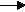gcsescience.com                                       4                                       gcsescience.com

Rates of Reaction

How can the Rate of the Reaction between
Calcium Carbonate and dilute Hydrochloric Acid be Measured?

HCl + calcium carbonatecalcium chloride + carbon dioxide + water.
2HCl(aq)   +   CaCO3(s)CaCl2(aq)     +      CO2(g)   +    H2O(l)

The rate of this reaction can be measured by looking at
the rate at which the product carbon dioxide gas is formed.

This can be done by having the reaction take place
in an open flask on an electric weighing machine
(a pan balance). As the carbon dioxide escapes to the air,
the mass of the flask and its contents will decrease.
You can take a reading from the balance every 30 seconds,
then plot a graph of loss of mass against time.

The gradient of the plot (the steepness of the slope)
shows the rate of the chemical reaction (how fast it is going).